Ch 5. Beam Deflections Multimedia Engineering Mechanics Integrationof Moment Integrationof Load Method ofSuperposition IndeterminateBeams
 Chapter 1. Stress/Strain 2. Torsion 3. Beam Shr/Moment 4. Beam Stresses 5. Beam Deflections 6. Beam-Advanced 7. Stress Analysis 8. Strain Analysis 9. Columns Appendix Basic Math Units Basic Equations Sections Material Properties Structural Shapes Beam Equations Search eBooks Dynamics Fluids Math Mechanics Statics Thermodynamics Author(s): Kurt Gramoll ©Kurt GramollMECHANICS - EXAMPLE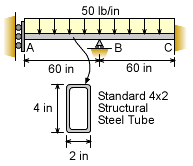Example

This example is the same problem as the case study previously solved. However, instead of using the principle of superposition, solve for point A deflection by integrating the load-deflection equation. The beam is a standard 4 x 2 structural steel tube. Assume Young 's modulus, E, is 29,000 ksi.

Solution

EIv´´´´ = -w(x)

This equation can be integrated four times, but four boundary conditions are required to determine the integration constants.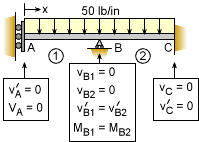Eight Boundary Conditions

The beam in this problem requires two sections due to the center support. This also means that there must be four boundary conditions. These conditions are listed in the diagram at the left.

Integrating the load-deflection equation for beam section one gives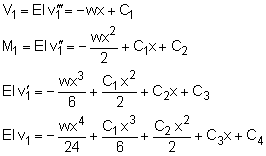For the second beam section, integration of the load-deflection equation gives,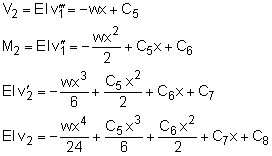Now each of the boundary conditions need to be applied which will produce eight equations. They are given below.

 No Condition 1) v1(x=0) = 0 2) V1(x=0) = 0 3) v1(x=60) = 0 4) v2(x=60) = 0 5) v2(x=120) = 0 6) v´2(x=120) = 0 7) v´1(x=60) = v´2(x=60) 8) M1(x=60) = M2(x=60)
Boundary Condition List

Boundary Condition 1)
0 = 0 + 0 + 0 + C3
C3 = 0

Boundary Condition 2)
0 = 0 + C1
C1 = 0

Boundary Condition 3)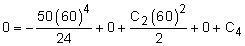27×106 = 1,800 C2 + C4

Boundary Condition 4)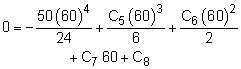27×106 = 36,000 C5 + 1,800 C6 + 60 C7 + C8

Boundary Condition 5)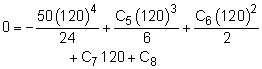432×106 = 288,000 C5 + 7,200 C6 + 120 C7 + C8

Boundary Condition 6)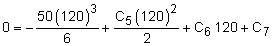14.4×106 = 7,200 C5 + 120 C6 + C7

Boundary Condition 7)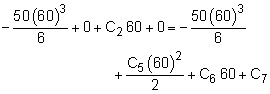0 = -60 C2 + 1,800 C5 + 60 C6 + C7

Boundary Condition 8)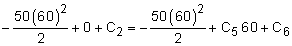0 = -C2 + 60 C5 + C6

The five equations from boundary conditions 4 through 8 can be used to solve for the 5 unknowns, C2, C5, C6, C7 and C8. These five equations are summarized in matrix form below.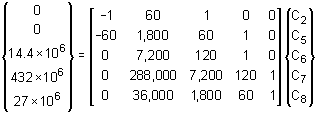It is difficult to solve these by hand but can easily solved with a matrix solver in most engineering calculators or with a spreadsheet. The solution for the unknowns are

C2= 39,000
C5= 5,400
C6= -285,000
C7 = 9,720,000
C8 = -237,600,000

Using boundary condition 3 gives

C4 = -43,200,000

All the integration constants are now known and can be used with the deflection equation. Young's Modulus is 29,000 ksi and the moment of inertia for the 4x2 steel tube is 5.32 in4 (from the Structural Shapes Appendix).

EI v1 = -wx4/24 + C1 x3/6 + C2 x2/2 + C3 x + C4

(29,000,000)(5.32) v1 = 0 + 0 + 0 + 0 - 43,200,000

v1 = 0.2800 in

This deflection at point A is the same as previously calculated using superposition.

Practice Homework and Test problems now available in the 'Eng Mechanics' mobile app
Includes over 400 problems with complete detailed solutions.
Available now at the Google Play Store and Apple App Store.